# Unit 3 Chemical Reactions Representing Chemical Reactions Chemical

• Slides: 7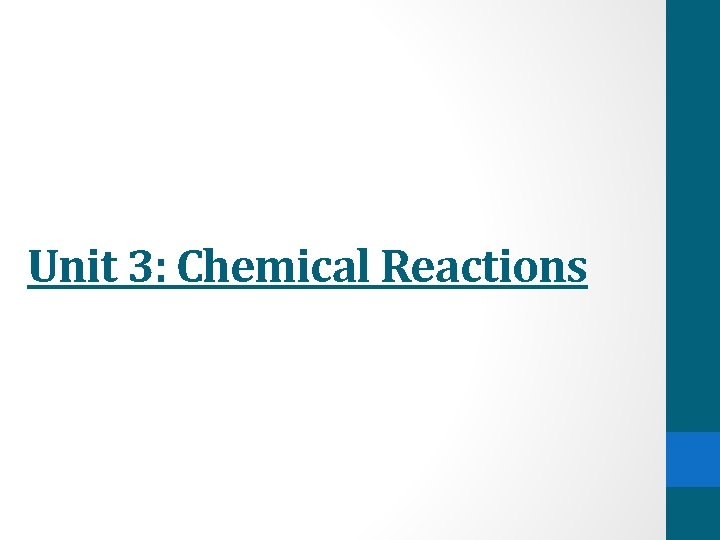Unit 3: Chemical ReactionsRepresenting Chemical Reactions • Chemical reactions occur when elements and compounds interact to form new substances. • A chemical equation is used to communicate what is occurring in a chemical reaction. • Word equations are used to represent a chemical reaction using only the names of the chemicals involved NOT the formulas. There are NO states in word equations.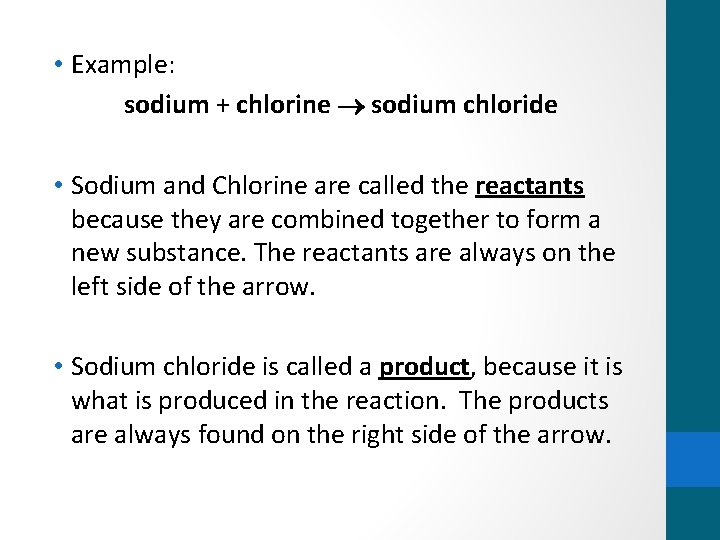• Example: sodium + chlorine sodium chloride • Sodium and Chlorine are called the reactants because they are combined together to form a new substance. The reactants are always on the left side of the arrow. • Sodium chloride is called a product, because it is what is produced in the reaction. The products are always found on the right side of the arrow.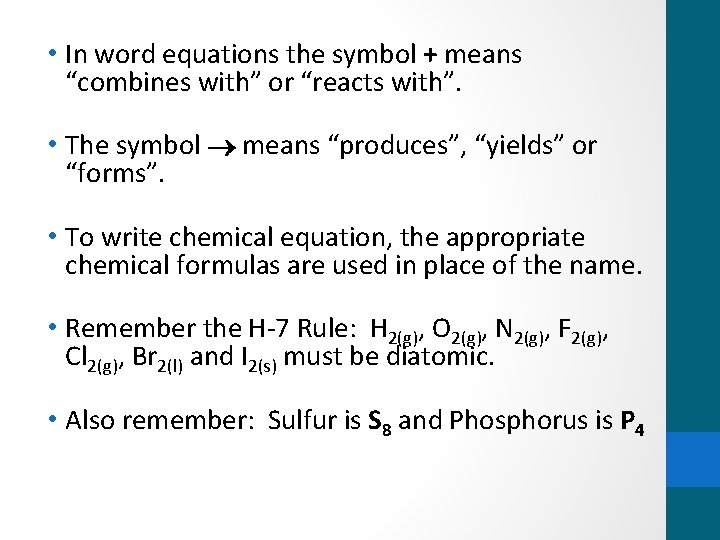• In word equations the symbol + means “combines with” or “reacts with”. • The symbol means “produces”, “yields” or “forms”. • To write chemical equation, the appropriate chemical formulas are used in place of the name. • Remember the H-7 Rule: H 2(g), O 2(g), N 2(g), F 2(g), Cl 2(g), Br 2(l) and I 2(s) must be diatomic. • Also remember: Sulfur is S 8 and Phosphorus is P 4• The Law of Conservation of Mass states that atoms cannot be created or destroyed. • Therefore, a chemical equation must be balanced, meaning there has to be an equal number of atoms of each element on both sides of the equation.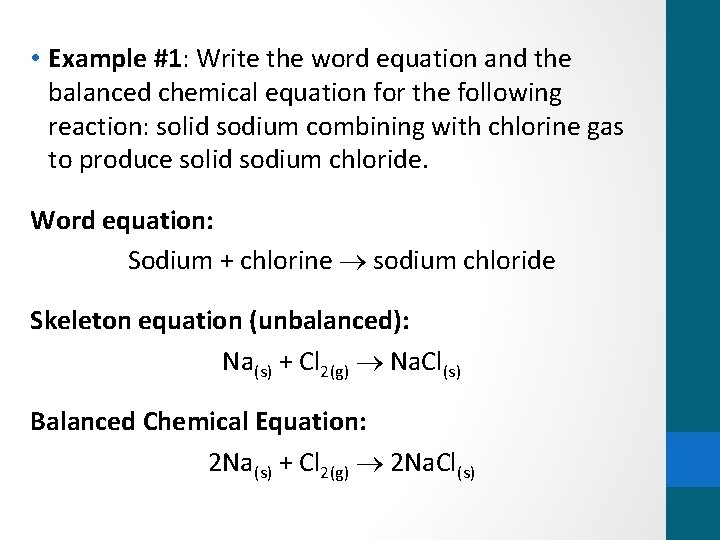• Example #1: Write the word equation and the balanced chemical equation for the following reaction: solid sodium combining with chlorine gas to produce solid sodium chloride. Word equation: Sodium + chlorine sodium chloride Skeleton equation (unbalanced): Na(s) + Cl 2(g) Na. Cl(s) Balanced Chemical Equation: 2 Na(s) + Cl 2(g) 2 Na. Cl(s)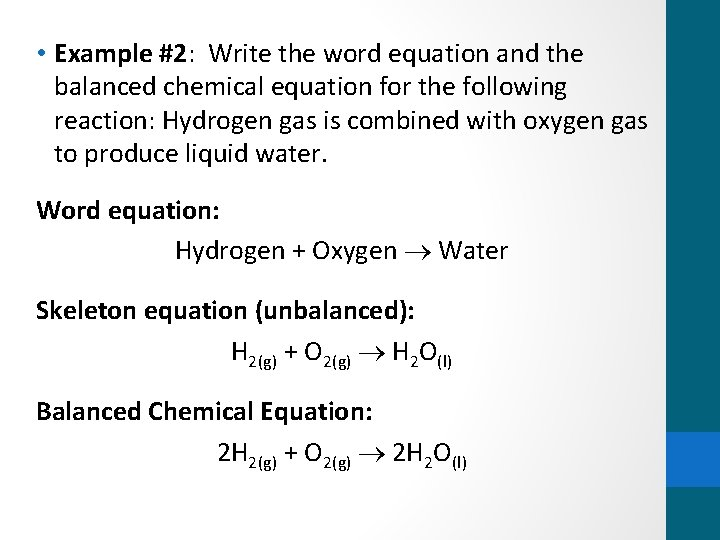• Example #2: Write the word equation and the balanced chemical equation for the following reaction: Hydrogen gas is combined with oxygen gas to produce liquid water. Word equation: Hydrogen + Oxygen Water Skeleton equation (unbalanced): H 2(g) + O 2(g) H 2 O(l) Balanced Chemical Equation: 2 H 2(g) + O 2(g) 2 H 2 O(l)